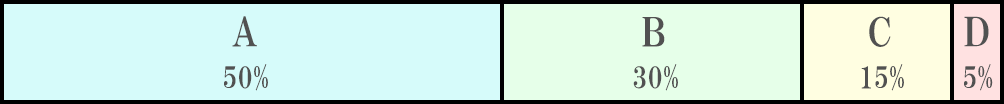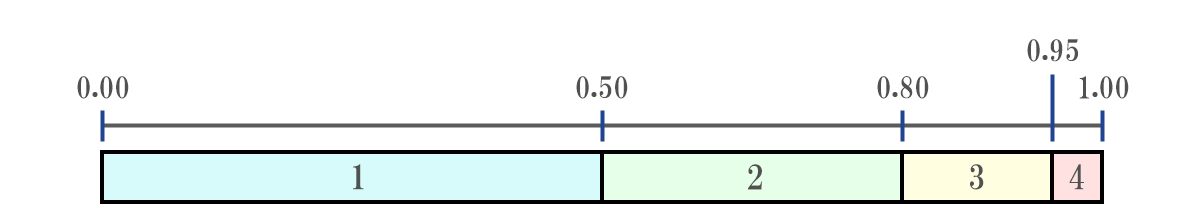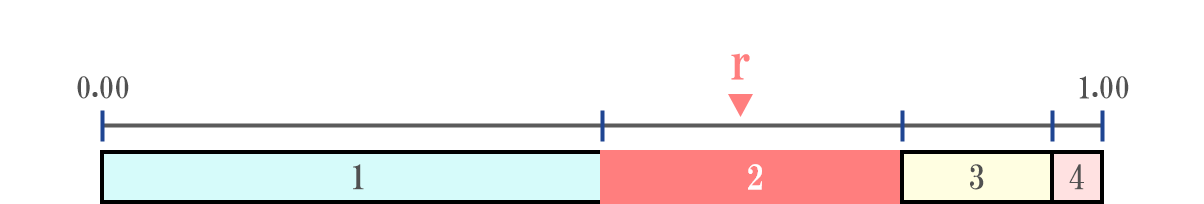# Overview

Given the following probability distribution, we would like to randomly determine one element according to this.The source code of the implementation is given at the end of the article. Since Unity etc. came up first as an operation destination, I decided to implement it in C #.
I don’t know if this feature has a name, but it’s called a gacha system because it resembles the gacha that has become popular in social games in recent years.

# Way of thinking

As input, we give a sequence of probability distributions \$ A_1, A_2, ⋯, A_N \$ consisting of \$ N \$ elements.

``````double[] odds = { 0.5, 0.3, 0.15, 0.05 };
``````First, determine one random real number \$ r \$ in the range of \$ [0, \ sum_ {i = 1} ^ {N} A_i] \$.

``````Random rnd = new Random();
double r = rnd.NextDouble();
``````Next, find the integer \$ k \$ such that \$ \ sum_ {i = 1} ^ {k} A_i \ leq r <\ sum_ {i = 1} ^ {k + 1} A_i \$.
A simple method to come up with is a linear search, but this time I will try to speed it up and implement it with a binary search. * The explanation of binary search is omitted.
Since the partial sum of the probability distribution must be calculated, the implementation as a whole is \$ O (N) \$. Not \$ O (\ log N) \$.

For the time being, define the signature of the function.
As arguments, take the probability distribution \$ A_1, A_2, ⋯, A_N \$, and the randomly determined value \$ r \; (0 \ leq r \ leq \ sum_ {i = 1} ^ {N} A_i) \$. ..
The return value should be the index \$ k \$ corresponding to \$ r \$.

``````public int BinarySearchInOdds(double[] odds, double r) {
//Returns the index corresponding to the value of r from the odds probability distribution
}
``````

The implementation of this part is not much different from the general binary search, so the explanation is omitted.
The idea is as above.

# Implementation

The `BinarySearchInOdds ()` method implements the “function that returns the index corresponding to the value of r from the probability distribution” that was omitted earlier.
You can easily draw a gacha by passing the probability distribution to the `Draw ()` method.
I wrote `Main ()` so that you can understand the specifications, so please take a look at the execution results as well.

``````using System;
using System.Collections.Generic;
using System.Linq;

public class Gacha {

//Returns the index of the element corresponding to r in the probability distribution
public static int BinarySearchInOdds(double[] odds, double r) {
if (r < 0) return -1;
if (odds.Length == 0) return -1;

//Obtain the partial sum of the probability distribution in advance
double[] sum_from_left = new double[odds.Length];
double[] sum_from_right = new double[odds.Length];
double odd_total = odds.Sum();
sum_from_left = 0;
sum_from_right = odd_total;
for (int i = 1; i < odds.Length; i++) {
sum_from_left[i] = sum_from_left[i-1] + odds[i-1];
sum_from_right[i] = sum_from_right[i-1] - odds[i-1];
}

int left = 0;
int right = odds.Length - 1;
int mid = left + (right - left) / 2;
double L_mid = sum_from_left[mid];

while (right >= left) {
double R_mid = L_mid + odds[mid];
if ((L_mid <= r && r < R_mid) || (r == R_mid && mid == odds.Length - 1)) {
//If the key is within the mid-th element
return mid;
} else if (r < L_mid) {
//If the key is in a range smaller than the range of the midth element
right = mid - 1;
mid = left + (right - left) / 2;
R_mid = L_mid - (odd_total - sum_from_left[mid + 1] - sum_from_right[right + 1]);
L_mid = R_mid - odds[mid];
} else {
//If the key is in a range larger than the range of the midth element
left = mid + 1;
mid = left + (right - left) / 2;
if (mid >= odds.Length) break;
L_mid = R_mid + (odd_total - sum_from_left[left] - sum_from_right[mid]);
R_mid = L_mid + odds[mid];
}
}
return -1;
}

//Returns the index of randomly determined elements according to the probability distribution
public static int Draw(double[] odds) {
Random rnd = new Random();
double r = rnd.NextDouble() * odds.Sum();
return BinarySearchInOdds(odds, r);
}

public static void Main() {
double[] odds1 = { 0.5, 3.0, 0, 2.0, 1.5, 0.1, 0.9 };
Console.WriteLine("odds1 = [" + string.Join(", ", odds1) + "]");
Console.WriteLine("r = 0.0  --->  " + BinarySearchInOdds(odds1, 0.0));
Console.WriteLine("r = 0.1  --->  " + BinarySearchInOdds(odds1, 0.1));
//When r is just on the border, the larger index is returned
Console.WriteLine("r = 0.5  --->  " + BinarySearchInOdds(odds1, 0.5));
Console.WriteLine("r = 2.0  --->  " + BinarySearchInOdds(odds1, 2.0));
Console.WriteLine("r = 4.0  --->  " + BinarySearchInOdds(odds1, 4.0));
Console.WriteLine("r = 6.0  --->  " + BinarySearchInOdds(odds1, 6.0));
Console.WriteLine("r = 7.08  --->  " + BinarySearchInOdds(odds1, 7.08));
Console.WriteLine("r = 7.7  --->  " + BinarySearchInOdds(odds1, 7.7));
//If r is equal to the sum of the probability distributions, the index of the last element is returned
Console.WriteLine("r = 8.0  --->  " + BinarySearchInOdds(odds1, 8.0));
//If r is out of range-1 is returned
Console.WriteLine("r = 10.0  --->  " + BinarySearchInOdds(odds1, 10.0));
//The same applies when r is negative
Console.WriteLine("r = -1.0  --->  " + BinarySearchInOdds(odds1, -1.0));
Console.WriteLine("");

double[] odds2 = { 1.0 };
Console.WriteLine("odds2 = [" + string.Join(", ", odds2) + "]");
//There is no problem even if there is only one element of the probability distribution
Console.WriteLine("r = 0.0  --->  " + BinarySearchInOdds(odds2, 0.0));
Console.WriteLine("r = 0.5  --->  " + BinarySearchInOdds(odds2, 0.5));
Console.WriteLine("r = 1.0  --->  " + BinarySearchInOdds(odds2, 1.0));
Console.WriteLine("");

double[] odds3 = {};
//If there is no element of probability distribution-1 is returned
Console.WriteLine("odds3 = [" + string.Join(", ", odds3) + "]");
Console.WriteLine("r = 0.0  --->  " + BinarySearchInOdds(odds3, 0.0));
Console.WriteLine("");

double[] odds4 = { 0.5, 0.35, 0.15, 0.05 };
Console.WriteLine("odds4 = [" + string.Join(", ", odds4) + "]");
//Draw a gacha 1 million times and check the emission probability
for (int i = 0; i < 1000000; i++) {
}
for (int i = 0; i < 4; i++) {
Console.WriteLine("element" + i + "  " + receivedCounts[i] + " / 1000000  ( " + receivedCounts[i] / 10000 + " %)");
}
}

}
``````

Execution result

``````odds1 = [0.5, 3, 0, 2, 1.5, 0.1, 0.9]
r = 0.0  --->  0
r = 0.1  --->  0
r = 0.5  --->  1
r = 2.0  --->  1
r = 4.0  --->  3
r = 6.0  --->  4
r = 7.08  --->  5
r = 7.7  --->  6
r = 8.0  --->  6
r = 10.0  --->  -1
r = -1.0  --->  -1

odds2 = 
r = 0.0  --->  0
r = 0.5  --->  0
r = 1.0  --->  0

odds3 = []
r = 0.0  --->  -1

odds4 = [0.5, 0.35, 0.15, 0.05]
Element 0 456796/ 1000000  ( 45 %)
Element 1 333225/ 1000000  ( 33 %)
Element 2 144646/ 1000000  ( 14 %)
Element 3 65333/ 1000000  ( 6 %)
``````

The execution speed was also measured.

``````N = 10^2  ->  2 ms
N = 10^3  ->  2 ms
N = 10^4  ->  2 ms
N = 10^5  ->  4 ms
N = 10^6  ->  18 ms
N = 10^7  ->  173 ms
N = 10^8  ->  1683 ms
``````

It seems that the order of processing time does not change until about \$ N = 10 ^ 5 \$. The speedup by binary search has come to life a little …

With this, you can always draw gacha.

Tags:

Updated: